## Predicting health issues for Senior Citizens based on “Realtime Weather Data” in Python

Hi Guys,

Today, I’ll be presenting a different kind of post here. I’ll be trying to predict health issues for senior citizens based on “realtime weather data” by blending open-source population data using some mock risk factor calculation. At the end of the post, I’ll be plotting these numbers into some graphs for better understanding.

Let’s drive!

For this first, we need realtime weather data. To do that, we need to subscribe to the data from OpenWeather API. For that, you have to register as a developer & you’ll receive a similar email from them once they have approved –

So, from the above picture, you can see that, you’ll be provided one API key & also offered a couple of useful API documentation. I would recommend exploring all the links before you try to use it.

You can also view your API key once you logged into their console. You can also create multiple API keys & the screen should look something like this –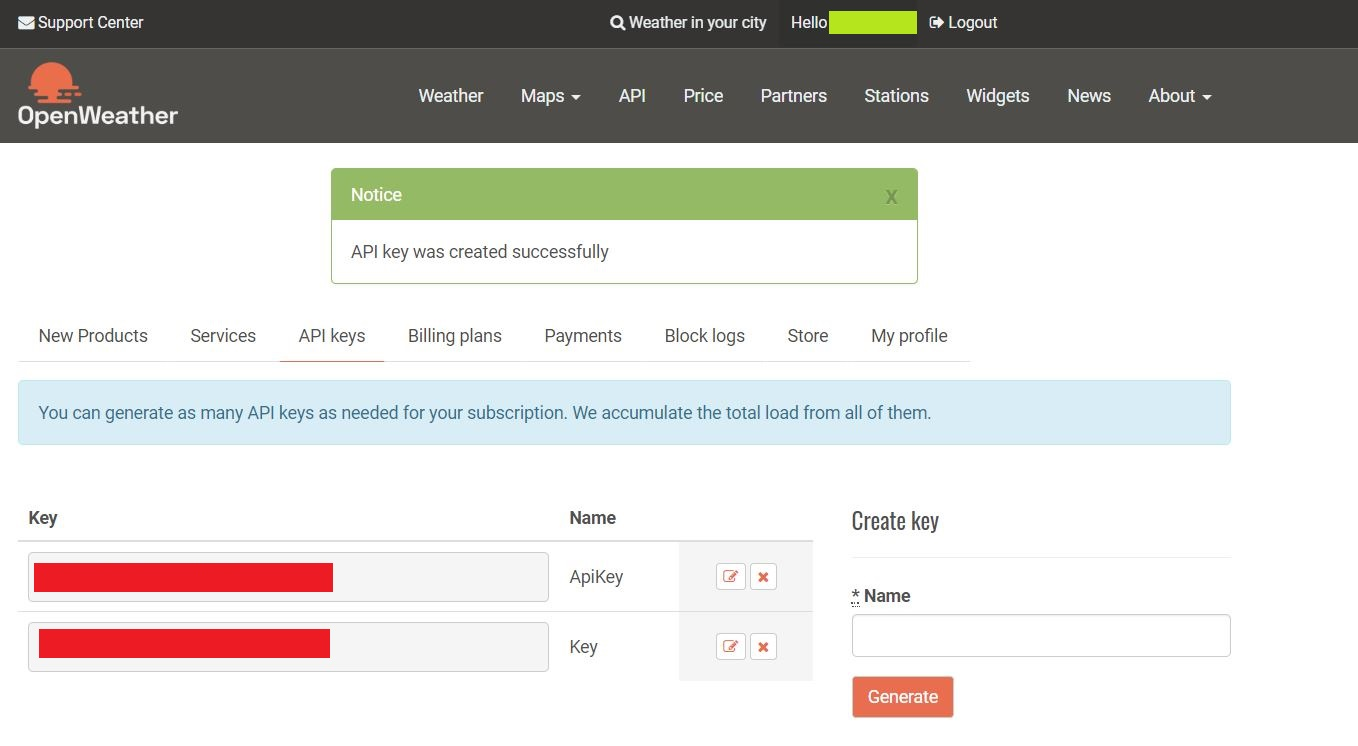For security reasons, I’ll be hiding my own keys & the same should be applicable for you as well.

I would say many of these free APIs might have some issues. So, I would recommend you to start testing the open API through postman before you jump into the Python development. Here is the glimpse of my test through the postman –

Once, I can see that the API is returning the result. I can work on it.

Apart from that, one needs to understand that these API might have limited use & also you need to know the consequences in terms of price & tier in case if you exceeded the limit. Here is the detail for this API –

For our demo, I’ll be using the Free tire only.

Let’s look into our other source data. We got the top 10 city population-wise over there internet. Also, we have collected sample Senior Citizen percentage against sex ratio across those cities. We have masked these values on top of that as this is just for education purposes.

1. CityDetails.csv

Here is the glimpse of this file –

So, this file only contains the total population across the top 10 cities in the USA.

2. SeniorCitizen.csv

This file contains the Sex ratio of Senior citizens across those top 10 cities by population.

Again, we are not going to discuss any script, which we’ve already discussed here.

Hence, we’re skipping clsL.py here.

1. clsConfig.py (This script contains all the parameters of the server.)

 ``` 1 2 3 4 5 6 7 8 9 10 11 12 13 14 15 16 17 18 19 20 21 22 23 24 25 26 27 28 29 30 31 32 33 34 35 36 37 38 39 40 41 42 43 44 45 46``` ```############################################## #### Written By: SATYAKI DE #### #### Written On: 19-Jan-2019 #### #### #### #### Objective: This script is a config #### #### file, contains all the keys for #### #### azure cosmos db. Application will #### #### process these information & perform #### #### various CRUD operation on Cosmos DB. #### ############################################## import os import platform as pl class clsConfig(object): Curr_Path = os.path.dirname(os.path.realpath(__file__)) os_det = pl.system() if os_det == "Windows": sep = '\\' else: sep = '/' config = { 'APP_ID': 1, 'URL': "http://api.openweathermap.org/data/2.5/weather", 'API_HOST': "api.openweathermap.org", 'API_KEY': "XXXXXXXXXXXXXXXXXXXXXX", 'API_TYPE': "application/json", 'CACHE': "no-cache", 'CON': "keep-alive", 'ARCH_DIR': Curr_Path + sep + 'arch' + sep, 'PROFILE_PATH': Curr_Path + sep + 'profile' + sep, 'LOG_PATH': Curr_Path + sep + 'log' + sep, 'REPORT_PATH': Curr_Path + sep + 'report', 'SRC_PATH': Curr_Path + sep + 'Src_File' + sep, 'APP_DESC_1': 'Open Weather Forecast', 'DEBUG_IND': 'N', 'INIT_PATH': Curr_Path, 'SRC_FILE': Curr_Path + sep + 'Src_File' + sep + 'CityDetails.csv', 'SRC_FILE_1': Curr_Path + sep + 'Src_File' + sep + 'SeniorCitizen.csv', 'SRC_FILE_INIT': 'CityDetails.csv', 'COL_LIST': ['base', 'all', 'cod', 'lat', 'lon', 'dt', 'feels_like', 'humidity', 'pressure', 'temp', 'temp_max', 'temp_min', 'name', 'country', 'sunrise', 'sunset', 'type', 'timezone', 'visibility', 'weather', 'deg', 'gust', 'speed'], 'COL_LIST_1': ['base', 'all', 'cod', 'lat', 'lon', 'dt', 'feels_like', 'humidity', 'pressure', 'temp', 'temp_max', 'temp_min', 'CityName', 'country', 'sunrise', 'sunset', 'type', 'timezone', 'visibility', 'deg', 'gust', 'speed', 'WeatherMain', 'WeatherDescription'], 'COL_LIST_2': ['CityName', 'Population', 'State'] } ```

2. clsWeather.py (This script contains the main logic to extract the realtime data from our subscribed weather API.)

 ``` 1 2 3 4 5 6 7 8 9 10 11 12 13 14 15 16 17 18 19 20 21 22 23 24 25 26 27 28 29 30 31 32 33 34 35 36 37 38 39 40 41 42 43 44 45 46 47 48 49 50 51 52 53 54 55 56 57 58 59 60 61``` ```############################################## #### Written By: SATYAKI DE #### #### Written On: 19-Jan-2020 #### #### Modified On 19-Jan-2020 #### #### #### #### Objective: Main scripts to invoke #### #### Indian Railway API. #### ############################################## import requests import logging import json from clsConfig import clsConfig as cf class clsWeather: def __init__(self): self.url = cf.config['URL'] self.openmapapi_host = cf.config['API_HOST'] self.openmapapi_key = cf.config['API_KEY'] self.openmapapi_cache = cf.config['CACHE'] self.openmapapi_con = cf.config['CON'] self.type = cf.config['API_TYPE'] def searchQry(self, rawQry): try: url = self.url openmapapi_host = self.openmapapi_host openmapapi_key = self.openmapapi_key openmapapi_cache = self.openmapapi_cache openmapapi_con = self.openmapapi_con type = self.type querystring = {"appid": openmapapi_key, "q": rawQry} print('Input JSON: ', str(querystring)) headers = { 'host': openmapapi_host, 'content-type': type, 'Cache-Control': openmapapi_cache, 'Connection': openmapapi_con } response = requests.request("GET", url, headers=headers, params=querystring) ResJson = response.text jdata = json.dumps(ResJson) ResJson = json.loads(jdata) return ResJson except Exception as e: ResJson = '' x = str(e) print(x) logging.info(x) ResJson = {'errorDetails': x} return ResJson ```

The key lines from this script –

```querystring = {"appid": openmapapi_key, "q": rawQry}

print('Input JSON: ', str(querystring))

'host': openmapapi_host,
'content-type': type,
'Cache-Control': openmapapi_cache,
'Connection': openmapapi_con
}

ResJson  = response.text```

In the above snippet, our application first preparing the payload & the parameters received from our param script. And then invoke the GET method to extract the real-time data in the form of JSON & finally sending the JSON payload to the primary calling function.

3. clsMap.py (This script contains the main logic to prepare the MAP using seaborn package & try to plot our custom made risk factor by blending the realtime data with our statistical data received over the internet.)

 ``` 1 2 3 4 5 6 7 8 9 10 11 12 13 14 15 16 17 18 19 20 21 22 23 24 25 26 27 28 29 30 31 32 33 34 35 36 37 38 39 40 41 42 43 44 45 46 47 48 49 50 51 52 53 54 55 56 57 58 59 60 61 62 63 64 65 66 67 68 69 70 71 72 73 74 75 76 77 78 79 80 81 82 83 84 85 86 87 88 89 90 91 92 93 94 95 96 97 98 99 100 101 102 103 104 105 106 107 108 109 110 111 112 113 114 115 116 117 118 119``` ```############################################## #### Written By: SATYAKI DE #### #### Written On: 19-Jan-2020 #### #### Modified On 19-Jan-2020 #### #### #### #### Objective: Main scripts to invoke #### #### plot into the Map. #### ############################################## import seaborn as sns import logging from clsConfig import clsConfig as cf import pandas as p import clsL as cl # This library requires later # to print the chart import matplotlib.pyplot as plt class clsMap: def __init__(self): self.src_file = cf.config['SRC_FILE_1'] def calculateRisk(self, row): try: # Let's assume some logic # 1. By default, 30% of Senior Citizen # prone to health Issue for each City # 2. Male Senior Citizen is 19% more prone # to illness than female. # 3. If humidity more than 70% or less # than 40% are 22% main cause of illness # 4. If feels like more than 280 or # less than 260 degree are 17% more prone # to illness. # Finally, this will be calculated per 1K # people around 10 blocks str_sex = str(row['Sex']) int_humidity = int(row['humidity']) int_feelsLike = int(row['feels_like']) int_population = int(str(row['Population']).replace(',','')) float_srcitizen = float(row['SeniorCitizen']) confidance_score = 0.0 SeniorCitizenPopulation = (int_population * float_srcitizen) if str_sex == 'Male': confidance_score = (SeniorCitizenPopulation * 0.30 * 0.19) + confidance_score else: confidance_score = (SeniorCitizenPopulation * 0.30 * 0.11) + confidance_score if ((int_humidity > 70) | (int_humidity < 40)): confidance_score = confidance_score + (int_population * 0.30 * float_srcitizen) * 0.22 if ((int_feelsLike > 280) | (int_feelsLike < 260)): confidance_score = confidance_score + (int_population * 0.30 * float_srcitizen) * 0.17 final_score = round(round(confidance_score, 2) / (1000 * 10), 2) return final_score except Exception as e: x = str(e) return x def setMap(self, dfInput): try: resVal = 0 df = p.DataFrame() debug_ind = 'Y' src_file = self.src_file # Initiating Log Class l = cl.clsL() df = dfInput # Creating a subset of desired columns dfMod = df[['CityName', 'temp', 'Population', 'humidity', 'feels_like']] l.logr('5.dfSuppliment.csv', debug_ind, dfMod, 'log') # Fetching Senior Citizen Data df = p.read_csv(src_file, index_col=False) # Merging two frames dfMerge = p.merge(df, dfMod, on=['CityName']) l.logr('6.dfMerge.csv', debug_ind, dfMerge, 'log') # Getting RiskFactor quotient from our custom made logic dfMerge['RiskFactor'] = dfMerge.apply(lambda row: self.calculateRisk(row), axis=1) l.logr('7.dfRiskFactor.csv', debug_ind, dfMerge, 'log') # Generating Map plotss # sns.lmplot(x='RiskFactor', y='SeniorCitizen', data=dfMerge, hue='Sex') # sns.lmplot(x='RiskFactor', y='SeniorCitizen', data=dfMerge, hue='Sex', markers=['o','v'], scatter_kws={'s':25}) sns.lmplot(x='RiskFactor', y='SeniorCitizen', data=dfMerge, col='Sex') # This is required when you are running # through normal Python & not through # Jupyter Notebook plt.show() return resVal except Exception as e: x = str(e) print(x) logging.info(x) resVal = x return resVal ```

Key lines from the above codebase –

```# Creating a subset of desired columns
dfMod = df[['CityName', 'temp', 'Population', 'humidity', 'feels_like']]

l.logr('5.dfSuppliment.csv', debug_ind, dfMod, 'log')

# Fetching Senior Citizen Data

# Merging two frames
dfMerge = p.merge(df, dfMod, on=['CityName'])

l.logr('6.dfMerge.csv', debug_ind, dfMerge, 'log')

# Getting RiskFactor quotient from our custom made logic
dfMerge['RiskFactor'] = dfMerge.apply(lambda row: self.calculateRisk(row), axis=1)

l.logr('7.dfRiskFactor.csv', debug_ind, dfMerge, 'log')```

Combining our Senior Citizen data with already processed data coming from our primary calling script. Also, here the application is calculating our custom logic to find out the risk factor figures. If you want to go through that, I’ve provided the logic to derive it. However, this is just a demo to find out similar figures. You should not rely on the logic that I’ve used (It is kind of my observation of life till now. :D).

The below lines are only required when you are running seaborn, not via Jupyter notebook.

`plt.show()`

4. callOpenMapWeatherAPI.py (This is the first calling script. This script also calls the realtime API & then blend the first file with it & pass the only relevant columns of data to our Map script to produce the graph.)

 ``` 1 2 3 4 5 6 7 8 9 10 11 12 13 14 15 16 17 18 19 20 21 22 23 24 25 26 27 28 29 30 31 32 33 34 35 36 37 38 39 40 41 42 43 44 45 46 47 48 49 50 51 52 53 54 55 56 57 58 59 60 61 62 63 64 65 66 67 68 69 70 71 72 73 74 75 76 77 78 79 80 81 82 83 84 85 86 87 88 89 90 91 92 93 94 95 96 97 98 99 100 101 102 103 104 105 106 107 108 109 110 111 112 113 114 115 116 117 118 119 120 121 122 123 124 125 126 127 128 129 130 131 132 133 134 135 136 137 138 139 140 141 142 143 144 145 146 147 148 149 150 151 152 153 154 155 156 157 158 159 160 161 162 163 164 165 166 167 168 169 170 171 172 173 174 175 176 177 178 179 180 181 182 183 184 185 186 187 188 189 190 191 192 193 194 195 196 197 198 199 200 201 202 203 204 205 206 207 208 209 210 211 212 213 214 215 216 217 218 219 220 221 222 223 224 225 226 227 228 229 230 231``` ```############################################## #### Written By: SATYAKI DE #### #### Written On: 19-Jan-2020 #### #### Modified On 19-Jan-2020 #### #### #### #### Objective: Main calling scripts. #### ############################################## from clsConfig import clsConfig as cf import pandas as p import clsL as cl import logging import datetime import json import clsWeather as ct import re import numpy as np import clsMap as cm # Disbling Warning def warn(*args, **kwargs): pass import warnings warnings.warn = warn # Lookup functions from # Azure cloud SQL DB var = datetime.datetime.now().strftime("%Y-%m-%d_%H-%M-%S") def getMainWeather(row): try: # Using regular expression to fetch time part only lkp_Columns = str(row['weather']) jpayload = str(lkp_Columns).replace("'", '"') #jpayload = json.dumps(lkp_Columns) payload = json.loads(jpayload) df_lkp = p.io.json.json_normalize(payload) df_lkp.columns = df_lkp.columns.map(lambda x: x.split(".")[-1]) str_main_weather = str(df_lkp.iloc['main']) return str_main_weather except Exception as e: x = str(e) str_main_weather = x return str_main_weather def getMainDescription(row): try: # Using regular expression to fetch time part only lkp_Columns = str(row['weather']) jpayload = str(lkp_Columns).replace("'", '"') #jpayload = json.dumps(lkp_Columns) payload = json.loads(jpayload) df_lkp = p.io.json.json_normalize(payload) df_lkp.columns = df_lkp.columns.map(lambda x: x.split(".")[-1]) str_description = str(df_lkp.iloc['description']) return str_description except Exception as e: x = str(e) str_description = x return str_description def main(): try: dfSrc = p.DataFrame() df_ret = p.DataFrame() ret_2 = '' debug_ind = 'Y' general_log_path = str(cf.config['LOG_PATH']) # Enabling Logging Info logging.basicConfig(filename=general_log_path + 'consolidatedIR.log', level=logging.INFO) # Initiating Log Class l = cl.clsL() # Moving previous day log files to archive directory arch_dir = cf.config['ARCH_DIR'] log_dir = cf.config['LOG_PATH'] col_list = cf.config['COL_LIST'] col_list_1 = cf.config['COL_LIST_1'] col_list_2 = cf.config['COL_LIST_2'] tmpR0 = "*" * 157 logging.info(tmpR0) tmpR9 = 'Start Time: ' + str(var) logging.info(tmpR9) logging.info(tmpR0) print("Archive Directory:: ", arch_dir) print("Log Directory::", log_dir) tmpR1 = 'Log Directory::' + log_dir logging.info(tmpR1) df2 = p.DataFrame() src_file = cf.config['SRC_FILE'] # Fetching data from source file df = p.read_csv(src_file, index_col=False) # Creating a list of City Name from the source file city_list = df['CityName'].tolist() # Declaring an empty dictionary merge_dict = {} merge_dict['city'] = df2 start_pos = 1 src_file_name = '1.' + cf.config['SRC_FILE_INIT'] for i in city_list: x1 = ct.clsWeather() ret_2 = x1.searchQry(i) # Capturing the JSON Payload res = json.loads(ret_2) # Converting dictionary to Pandas Dataframe # df_ret = p.read_json(ret_2, orient='records') df_ret = p.io.json.json_normalize(res) df_ret.columns = df_ret.columns.map(lambda x: x.split(".")[-1]) # Removing any duplicate columns df_ret = df_ret.loc[:, ~df_ret.columns.duplicated()] # l.logr(str(start_pos) + '.1.' + src_file_name, debug_ind, df_ret, 'log') start_pos = start_pos + 1 # If all the conversion successful # you won't get any gust column # from OpenMap response. Hence, we # need to add dummy reason column # to maintain the consistent structures if 'gust' not in df_ret.columns: df_ret = df_ret.assign(gust=999999)[['gust'] + df_ret.columns.tolist()] # Resetting the column orders as per JSON column_order = col_list df_mod_ret = df_ret.reindex(column_order, axis=1) if start_pos == 1: merge_dict['city'] = df_mod_ret else: d_frames = [merge_dict['city'], df_mod_ret] merge_dict['city'] = p.concat(d_frames) start_pos += 1 for k, v in merge_dict.items(): l.logr(src_file_name, debug_ind, merge_dict[k], 'log') # Now opening the temporary file temp_log_file = log_dir + src_file_name dfNew = p.read_csv(temp_log_file, index_col=False) # Extracting Complex columns dfNew['WeatherMain'] = dfNew.apply(lambda row: getMainWeather(row), axis=1) dfNew['WeatherDescription'] = dfNew.apply(lambda row: getMainDescription(row), axis=1) l.logr('2.dfNew.csv', debug_ind, dfNew, 'log') # Removing unwanted columns & Renaming key columns dfNew.drop(['weather'], axis=1, inplace=True) dfNew.rename(columns={'name': 'CityName'}, inplace=True) l.logr('3.dfNewMod.csv', debug_ind, dfNew, 'log') # Now joining with the main csv # to get the complete picture dfMain = p.merge(df, dfNew, on=['CityName']) l.logr('4.dfMain.csv', debug_ind, dfMain, 'log') # Let's extract only relevant columns dfSuppliment = dfMain[['CityName', 'Population', 'State', 'country', 'feels_like', 'humidity', 'pressure', 'temp', 'temp_max', 'temp_min', 'visibility', 'deg', 'gust', 'speed', 'WeatherMain', 'WeatherDescription']] l.logr('5.dfSuppliment.csv', debug_ind, dfSuppliment, 'log') # Let's pass this to our map section x2 = cm.clsMap() ret_3 = x2.setMap(dfSuppliment) if ret_3 == 0: print('Successful Map Generated!') else: print('Please check the log for further issue!') print("-" * 60) print() print('Finding Story points..') print("*" * 157) logging.info('Finding Story points..') logging.info(tmpR0) tmpR10 = 'End Time: ' + str(var) logging.info(tmpR10) logging.info(tmpR0) except ValueError as e: print(str(e)) print("No relevant data to proceed!") logging.info("No relevant data to proceed!") except Exception as e: print("Top level Error: args:{0}, message{1}".format(e.args, e.message)) if __name__ == "__main__": main() ```

Key snippet from the above script –

```# Capturing the JSON Payload

# Converting dictionary to Pandas Dataframe
df_ret = p.io.json.json_normalize(res)
df_ret.columns = df_ret.columns.map(lambda x: x.split(".")[-1])```

Once the application received the JSON response from the realtime API, the application is converting it to pandas dataframe.

```# Removing any duplicate columns
df_ret = df_ret.loc[:, ~df_ret.columns.duplicated()]```

Since this is a complex JSON response. The application might encounter duplicate columns, which might cause a problem later. Hence, our app is removing all these duplicate columns as they are not required for our cases.

```if 'gust' not in df_ret.columns:
df_ret = df_ret.assign(gust=999999)[['gust'] + df_ret.columns.tolist()]```

There is a possibility that the application might not receive all the desired attributes from the realtime API. Hence, the above lines will check & add a dummy column named gust for those records in case if they are not present in the JSON response.

```if start_pos == 1:
merge_dict['city'] = df_mod_ret
else:
d_frames = [merge_dict['city'], df_mod_ret]
merge_dict['city'] = p.concat(d_frames)```

These few lines required as our API has a limitation of responding with only one city at a time. Hence, in this case, we’re retrieving one town at a time & finally merge them into a single dataframe before creating a temporary source file for the next step.

At this moment our data should look like this –

Let’s check the weather column. We need to extract the main & description for our dashboard, which will be coming in the next installment.

```# Extracting Complex columns
dfNew['WeatherMain'] = dfNew.apply(lambda row: getMainWeather(row), axis=1)
dfNew['WeatherDescription'] = dfNew.apply(lambda row: getMainDescription(row), axis=1)```

Hence, we’ve used the following two functions to extract these values & the critical snippet from one of the service is as follows –

```lkp_Columns = str(row['weather'])

df_lkp.columns = df_lkp.columns.map(lambda x: x.split(".")[-1])

str_main_weather = str(df_lkp.iloc['main'])```

The above lines extracting the weather column & replacing the single quotes with the double quotes before the application is trying to convert that to JSON. Once it converted to JSON, the json_normalize will easily serialize it & create individual columns out of it. Once you have them captured inside the pandas dataframe, you can extract the unique values & store them & return them to your primary calling function.

```# Let's pass this to our map section
x2 = cm.clsMap()
ret_3 = x2.setMap(dfSuppliment)

if ret_3 == 0:
print('Successful Map Generated!')
else:
print('Please check the log for further issue!')```

In the above lines, the application will invoke the Map class to calculate the remaining logic & then plotting the data into the seaborn graph.

Let’s just briefly see the central directory structure –

Here is the log directory –

And, finally, the source directory should look something like this –

Now, let’s runt the application –

Following lines are essential –

`sns.lmplot(x='RiskFactor', y='SeniorCitizen', data=dfMerge, hue='Sex')`

This will project the plot like this –

Or,

`sns.lmplot(x='RiskFactor', y='SeniorCitizen', data=dfMerge, hue='Sex', markers=['o','v'], scatter_kws={'s':25})`

This will lead to the following figures –

As you can see, here, using the marker of (‘o’/’v’) leads to two different symbols for the different gender.

Or,

`sns.lmplot(x='RiskFactor', y='SeniorCitizen', data=dfMerge, col='Sex')`

So, in this case, the application has created two completely different sets for Sex.

So, finally, we’ve done it. 😀

In the next post, I’ll be doing some more improvisation on top of these data sets. Till then – Happy Avenging! 🙂

Note: All the data posted here are representational data & available over the internet & for educational purpose only.

## The advanced concept of Pandas & Numpy with an aggregate & lookup of file logging (A crossover over of Space Stone & Soul Stone from the Python verse)

Today, we’ll be implementing the advanced concept of Pandas & Numpy & how one can aggregate data & produce meaningful data insights into your business, which makes an impact on your overall profit.

First, let us understand the complexity of the problem & what we’re looking to achieve here. For that, you need to view the source data & lookup data & how you want to process the data.

##### Source Data:The above picture is a sample data-set from a Bank (Data available on U.S public forum), which captures the information of the customer’s current account balance. Let’s look into the look-up files sample data –

##### First File:##### Second File: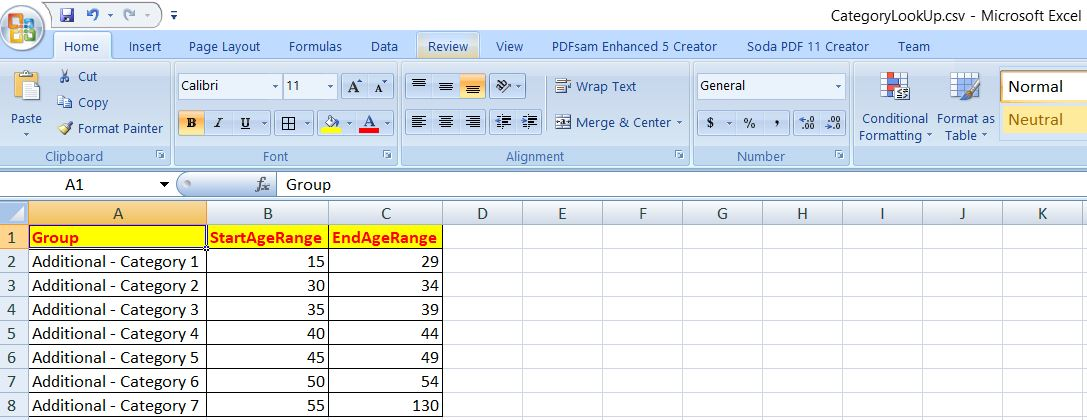So, one can clearly see, Bank is trying to get a number of stories based on the existing data.

#### Challenges:

The first lookup file contains data in a manner where the column of our source file is row here. Hence, you need to somehow bring the source data as per the lookup file to get the other relevant information & then joining that with the second lookup file to bring all the data point for your storyline.

Look-Up Configuration:

In order to match the look-up data with our source data, we’ll be adding two new columns, which will help the application to process the correct row out of the entries provided in the look-up file 1.As you can see from the above picture, that two new columns i.e. Category & Stat have added in this context. Here, the category contains metadata information. If a column has a significant number of unique values, then we’re marking it as ‘D in the category. In this case, the bank doesn’t offer any scheme based on the customer’s name. Hence, these fields are marked with ‘I. For the Gender column, the application has less number of unique records i.e. either ‘Male‘ or ‘Female‘. As a result, we provided two corresponding entries. Remember, DateJoined is a key column here. Even though we marked its category as ‘I‘, which denote no transformation requires – ‘K‘ will denote that it is the driving column apart from one of the surrogate key [PKEY] that we’ll be generating during our application transformation process. I’ll discuss that in the respective snippet discussion.

#### Our Goal:

Based on the source data, We need to find the following story & published that in an excel sheet separately.

1. The country, Gender wise Bank’s contribution.
2. The country, Job-wise Bank’s contribution.
3. The country & Age range wise Saving trends & Bank’s contribution.

#### A little note on Bank’s Contribution:

Let us explain, what exactly means by Bank’s contribution. Sometimes, bank want’s to encourage savings to an individual client based on all the available factors. So, let’s assume that – Bank contribute \$1 for every \$150 saving of a person. Again this \$1 may vary based on the Age Range & gender to promote a specific group. Also, when someone opens any savings account with the bank, by default bank contributed a sum of \$100 at the time when they open an account for a short period of time as part of their promotion strategy. These details you will get it from first lookup file. Second lookup file contains the age range category base on the Group that is available in First Lookup file.

#### Python Scripts:

In this installment, we’ll be reusing the following python scripts, which is already discussed in my earlier post

• clsFindFile.py
• clsL.py

So, I’m not going to discuss these scripts.

1. clsParam.py (This script will create the split csv files or final merge file after the corresponding process. However, this can be used as normal verbose debug logging as well. Hence, the name comes into the picture.)

 ``` 1 2 3 4 5 6 7 8 9 10 11 12 13 14 15 16 17 18 19 20 21 22 23 24 25 26 27 28 29 30 31 32``` ```########################################### #### Written By: SATYAKI DE ######## #### Written On: 04-Apr-2019 ######## ########################################### import os import platform as pl class clsParam(object): os_det = pl.system() dir_sep = '' if os_det == "Windows": dir_sep = "\\" else: dir_sep = '/' config = { 'MAX_RETRY' : 5, 'PATH' : os.path.dirname(os.path.realpath(__file__)) + dir_sep, 'SRC_DIR' : os.path.dirname(os.path.realpath(__file__)) + dir_sep + 'src_files' + dir_sep, 'FIN_DIR': os.path.dirname(os.path.realpath(__file__)) + dir_sep + 'finished' + dir_sep, 'LKP_DIR': os.path.dirname(os.path.realpath(__file__)) + dir_sep + 'lkp_files' + dir_sep, 'LOG_DIR': os.path.dirname(os.path.realpath(__file__)) + dir_sep + 'log' + dir_sep, 'LKP_FILE': 'DataLookUp', 'LKP_CATG_FILE': 'CategoryLookUp', 'LKP_FILE_DIR_NM': 'lkp_files', 'SRC_FILE_DIR_NM': 'src_files', 'FIN_FILE_DIR_NM': 'finished', 'LOG_FILE_DIR_NM': 'log', 'DEBUG_IND': 'Y' } ```

2. clsLookUpDataRead.py (This script will look into the lookup file & this will generate the combined lookup result as we’ve two different lookup files. Hence, the name comes into the picture.)

 ``` 1 2 3 4 5 6 7 8 9 10 11 12 13 14 15 16 17 18 19 20 21 22 23 24 25 26 27 28 29 30 31 32 33 34 35 36 37 38 39 40 41 42 43 44 45 46 47 48 49 50 51 52 53 54 55 56 57 58 59 60 61 62 63 64 65 66 67 68 69 70 71 72 73 74 75 76 77 78 79 80 81 82 83 84 85 86 87 88 89 90 91 92 93 94 95 96 97 98 99 100 101 102 103 104 105 106 107 108 109 110 111 112 113 114 115 116 117 118 119 120 121 122 123 124 125 126 127 128 129``` ```########################################### #### Written By: SATYAKI DE ######## #### Written On: 04-Apr-2019 ######## ########################################### import pandas as p import clsFindFile as c import clsL as log from clsParam import clsParam as cf import datetime # Disbling Warnings def warn(*args, **kwargs): pass import warnings warnings.warn = warn class clsLookUpDataRead(object): def __init__(self, lkpFilename): self.lkpFilename = lkpFilename self.lkpCatgFilename = cf.config['LKP_CATG_FILE'] self.path = cf.config['PATH'] self.subdir = str(cf.config['LOG_FILE_DIR_NM']) # To disable logging info self.Ind = cf.config['DEBUG_IND'] self.var = datetime.datetime.now().strftime(".%H.%M.%S") def getNaN2Null(self, row): try: str_val = '' str_val = str(row['Group']).replace('nan', '').replace('NaN','') return str_val except: str_val = '' return str_val def ReadTable(self): # Assigning Logging Info lkpF = [] lkpF_2 = [] var = self.var Ind = self.Ind subdir = self.subdir # Initiating Logging Instances clog = log.clsL() try: # Assinging Lookup file name lkpFilename = self.lkpFilename # Fetching the actual look-up file name f = c.clsFindFile(lkpFilename, str(cf.config['LKP_FILE_DIR_NM'])) lkp_file_list = list(f.find_file()) # Ideally look-up will be only one file # Later it will be converted to table for i in range(len(lkp_file_list)): lkpF = lkp_file_list[i] # Fetching the content of the look-up file df_lkpF = p.read_csv(lkpF, index_col=False) # Fetching Category LookUp File LkpCatgFileName = self.lkpCatgFilename f1 = c.clsFindFile(LkpCatgFileName, str(cf.config['LKP_FILE_DIR_NM'])) lkp_file_list_2 = list(f1.find_file()) # Ideally look-up will be only one file # Later it will be converted to table for j in range(len(lkp_file_list_2)): lkpF_2 = lkp_file_list_2[j] # Fetching the content of the look-up file df_lkpF_2 = p.read_csv(lkpF_2, index_col=False) # Changing both the column data type as same type df_lkpF['Group_1'] = df_lkpF['Group'].astype(str) df_lkpF_2['Group_1'] = df_lkpF_2['Group'].astype(str) # Dropping the old column df_lkpF.drop(['Group'], axis=1, inplace=True) df_lkpF_2.drop(['Group'], axis=1, inplace=True) # Renaming the changed data type column with the old column name df_lkpF.rename(columns={'Group_1':'Group'}, inplace=True) df_lkpF_2.rename(columns={'Group_1': 'Group'}, inplace=True) # Merging two lookup dataframes to form Final Consolidated Dataframe df_Lkp_Merge = p.merge( df_lkpF[['TableName', 'ColumnOrder', 'ColumnName', 'MappedColumnName', 'Category', 'Stat', 'Group', 'BankContribution']], df_lkpF_2[['StartAgeRange', 'EndAgeRange', 'Group']], on=['Group'], how='left') # Converting NaN to Nul or empty string df_Lkp_Merge['GroupNew'] = df_Lkp_Merge.apply(lambda row: self.getNaN2Null(row), axis=1) # Dropping the old column & renaming the new column df_Lkp_Merge.drop(['Group'], axis=1, inplace=True) df_Lkp_Merge.rename(columns={'GroupNew': 'Group'}, inplace=True) clog.logr('1.df_Lkp_Merge' + var + '.csv', Ind, df_Lkp_Merge, subdir) return df_Lkp_Merge except(FileNotFoundError, IOError) as s: y = str(s) print(y) # Declaring Empty Dataframe df_error = p.DataFrame() return df_error except Exception as e: x = str(e) print(x) # Declaring Empty Dataframe df_error = p.DataFrame() return df_error ```

Key lines from this script –

`# Fetching the actual look-up file namef = c.clsFindFile(lkpFilename, str(cf.config['LKP_FILE_DIR_NM']))lkp_file_list = list(f.find_file())# Ideally look-up will be only one file# Later it will be converted to tablefor i in range(len(lkp_file_list)):    lkpF = lkp_file_list[i]# Fetching the content of the look-up filedf_lkpF = p.read_csv(lkpF, index_col=False)`

Here, the application will try to find out the lookup file based on the file name pattern & directory path. And, then load the data into the dataframe.

`# Fetching Category LookUp FileLkpCatgFileName = self.lkpCatgFilenamef1 = c.clsFindFile(LkpCatgFileName, str(cf.config['LKP_FILE_DIR_NM']))lkp_file_list_2 = list(f1.find_file())# Ideally look-up will be only one file# Later it will be converted to tablefor j in range(len(lkp_file_list_2)):    lkpF_2 = lkp_file_list_2[j]# Fetching the content of the look-up filedf_lkpF_2 = p.read_csv(lkpF_2, index_col=False)`

In this step, the second lookup file will be loaded into the second dataframe.

`# Changing both the column data type as same typedf_lkpF['Group_1'] = df_lkpF['Group'].astype(str)df_lkpF_2['Group_1'] = df_lkpF_2['Group'].astype(str)# Dropping the old columndf_lkpF.drop(['Group'], axis=1, inplace=True)df_lkpF_2.drop(['Group'], axis=1, inplace=True)# Renaming the changed data type column with the old column namedf_lkpF.rename(columns={'Group_1':'Group'}, inplace=True)df_lkpF_2.rename(columns={'Group_1': 'Group'}, inplace=True)`

It is always better to cast the same datatype for those columns, which will be used part of the joining key. The above snippet does exactly that.

`# Merging two lookup dataframes to form Final Consolidated Dataframedf_Lkp_Merge = p.merge(                        df_lkpF[['TableName', 'ColumnOrder', 'ColumnName', 'MappedColumnName',                                 'Category', 'Stat', 'Group', 'BankContribution']],                        df_lkpF_2[['StartAgeRange', 'EndAgeRange', 'Group']],                        on=['Group'], how='left')`

In this step, the first lookup file will be left join with the second lookup file based on Group column.

`# Converting NaN to Nul or empty stringdf_Lkp_Merge['GroupNew'] = df_Lkp_Merge.apply(lambda row: self.getNaN2Null(row), axis=1)# Dropping the old column & renaming the new columndf_Lkp_Merge.drop(['Group'], axis=1, inplace=True)df_Lkp_Merge.rename(columns={'GroupNew': 'Group'}, inplace=True)`

Once merge is done, key columns need to suppress ‘NaN’ values to Null for better data process.

3. clsPivotLookUp.py (This script will actually contain the main logic to process & merge the data between source & lookup files & create group data & based on that data point will be produced & captured in the excel. Hence, the name comes into the picture.)

 ``` 1 2 3 4 5 6 7 8 9 10 11 12 13 14 15 16 17 18 19 20 21 22 23 24 25 26 27 28 29 30 31 32 33 34 35 36 37 38 39 40 41 42 43 44 45 46 47 48 49 50 51 52 53 54 55 56 57 58 59 60 61 62 63 64 65 66 67 68 69 70 71 72 73 74 75 76 77 78 79 80 81 82 83 84 85 86 87 88 89 90 91 92 93 94 95 96 97 98 99 100 101 102 103 104 105 106 107 108 109 110 111 112 113 114 115 116 117 118 119 120 121 122 123 124 125 126 127 128 129 130 131 132 133 134 135 136 137 138 139 140 141 142 143 144 145 146 147 148 149 150 151 152 153 154 155 156 157 158 159 160 161 162 163 164 165 166 167 168 169 170 171 172 173 174 175 176 177 178 179 180 181 182 183 184 185 186 187 188 189 190 191 192 193 194 195 196 197 198 199 200 201 202 203 204 205 206 207 208 209 210 211 212 213 214 215 216 217 218 219 220 221 222 223 224 225 226 227 228 229 230 231 232 233 234 235 236 237 238 239 240 241 242 243 244 245 246 247 248 249 250 251 252 253 254 255 256 257 258 259 260 261 262 263 264 265 266 267 268 269 270 271 272 273 274 275 276 277 278 279 280 281 282 283 284 285 286 287 288 289 290 291 292 293 294 295 296 297 298 299 300 301 302 303 304 305 306 307 308 309 310 311 312 313 314 315 316 317 318 319 320 321 322 323 324 325 326 327 328 329 330 331 332 333 334 335 336 337 338 339 340 341 342 343 344 345 346 347 348 349 350 351 352 353 354 355 356 357 358 359 360 361 362 363 364 365 366 367 368 369 370 371 372 373 374 375 376 377 378 379 380 381 382 383 384 385 386 387 388 389 390 391 392 393 394 395 396 397 398 399 400 401 402 403 404 405 406 407 408 409 410 411 412 413 414 415 416 417 418 419 420 421 422 423 424 425 426 427 428 429 430 431 432 433 434 435 436 437 438 439 440 441 442 443 444 445 446 447 448 449 450 451 452 453 454 455 456 457 458 459 460 461 462 463 464 465 466 467 468 469 470 471 472 473 474 475 476 477 478 479 480 481 482 483 484 485 486 487 488 489 490 491 492 493 494 495 496 497 498 499 500 501 502 503 504 505``` ```########################################### #### Written By: SATYAKI DE ######## #### Written On: 04-Apr-2019 ######## ########################################### import pandas as p import numpy as np import clsFindFile as c import clsL as log import datetime from clsParam import clsParam as cf from pandas import ExcelWriter # Disbling Warnings def warn(*args, **kwargs): pass import warnings warnings.warn = warn class clsPivotLookUp(object): def __init__(self, srcFilename, tgtFileName, df_lkpF): self.srcFilename = srcFilename self.tgtFileName = tgtFileName self.df_lkpF = df_lkpF self.lkpCatgFilename = cf.config['LKP_CATG_FILE'] self.path = cf.config['PATH'] self.subdir = str(cf.config['LOG_FILE_DIR_NM']) self.subdir_2 = str(cf.config['FIN_FILE_DIR_NM']) # To disable logging info self.Ind = cf.config['DEBUG_IND'] self.report_path = cf.config['FIN_DIR'] def dfs_tabs(self, df_list, sheet_list, file_name): try: cnt = 0 number_rows = 0 writer = p.ExcelWriter(file_name, engine='xlsxwriter') for dataframe, sheet in zip(df_list, sheet_list): number_rows = int(dataframe.shape) number_cols = int(dataframe.shape) if cnt == 0: dataframe.to_excel(writer, sheet_name=sheet, startrow=7, startcol=5) else: dataframe.to_excel(writer, sheet_name=sheet, startrow=5, startcol=0) # Get the xlsxwriter workbook & worksheet objects workbook = writer.book worksheet = writer.sheets[sheet] worksheet.set_zoom(90) if cnt == 0: worksheet.set_column('A:E', 4) worksheet.set_column('F:F', 20) worksheet.set_column('G:G', 10) worksheet.set_column('H:J', 20) # Insert an Image worksheet.insert_image('E1', 'Logo.png', {'x_scale':0.6, 'y_scale':0.8}) # Add a number format for cells with money. money_fmt = workbook.add_format({'num_format': '\$#,##0', 'border': 1}) worksheet.set_column('H:H', 20, money_fmt) # Define our range for color formatting color_range = "F9:F{}".format(number_rows * 2 + 1) # Add a format. Red fill with the dark red text red_format = workbook.add_format({'bg_color':'#FEC7CE', 'font_color':'#0E0E08', 'border':1}) # Add a format. Green fill with the dark green text green_format = workbook.add_format({'bg_color': '#D0FCA4', 'font_color': '#0E0E08', 'border': 1}) # Add a format. Cyan fill with the dark green text mid_format = workbook.add_format({'bg_color': '#6FC2D8', 'font_color': '#0E0E08', 'border': 1}) # Add a format. Other fill with the dark green text oth_format = workbook.add_format({'bg_color': '#AFC2D8', 'font_color': '#0E0E08', 'border': 1}) worksheet.conditional_format(color_range, {'type':'cell', 'criteria':'equal to', 'value':'"England"', 'format': green_format}) worksheet.conditional_format(color_range, {'type': 'cell', 'criteria': 'equal to', 'value': '"Northern Ireland"', 'format': mid_format}) worksheet.conditional_format(color_range, {'type': 'cell', 'criteria': 'equal to', 'value': '"Scotland"', 'format': red_format}) worksheet.conditional_format(color_range, {'type': 'cell', 'criteria': 'equal to', 'value': '"Wales"', 'format': oth_format}) else: first_row = 5 first_col = 0 last_row = first_row + (number_rows * 2) last_col = number_cols - 1 if cnt == 1: worksheet.set_column('A:D', 20) else: worksheet.set_column('A:E', 20) worksheet.set_column('F:F', 20) # Add a number format for cells with money. # money_fmt = workbook.add_format({'num_format': '\$#,##0', 'bold': True, 'border':1}) money_fmt = workbook.add_format({'num_format': '\$#,##0', 'border': 1}) # Amount columns if cnt == 1: worksheet.set_row(6, 0, money_fmt) worksheet.set_column('C:C', 20, money_fmt) else: worksheet.set_row(6, 0, money_fmt) worksheet.set_column('D:F', 20, money_fmt) # Insert an Image worksheet.insert_image('B1', 'Logo.png', {'x_scale': 0.5, 'y_scale': 0.5}) # Add a format. Red fill with the dark red text red_format = workbook.add_format({'bg_color': '#FEC7CE', 'font_color': '#0E0E08'}) # Add a format. Green fill with the dark green text green_format = workbook.add_format({'bg_color': '#D0FCA4', 'font_color': '#0E0E08'}) # Add a format. Cyan fill with the dark green text mid_format = workbook.add_format({'bg_color': '#6FC2D8', 'font_color': '#0E0E08'}) # Add a format. Other fill with the dark green text oth_format = workbook.add_format({'bg_color': '#AFC2D8', 'font_color': '#0E0E08'}) # Fill colour based on formula worksheet.conditional_format(first_row, first_col, last_row, last_col, {'type': 'formula', 'criteria': '=INDIRECT("A"&ROW())="England"', 'format': green_format}) worksheet.conditional_format(first_row, first_col, last_row, last_col, {'type': 'formula', 'criteria': '=INDIRECT("A"&ROW())="Northern Ireland"', 'format': mid_format}) worksheet.conditional_format(first_row, first_col, last_row, last_col, {'type': 'formula', 'criteria': '=INDIRECT("A"&ROW())="Scotland"', 'format': red_format}) worksheet.conditional_format(first_row, first_col, last_row, last_col, {'type': 'formula', 'criteria': '=INDIRECT("A"&ROW())="Wales"', 'format': oth_format}) cnt += 1 writer.save() writer.close() return 0 except Exception as e: x = str(e) print(x) return 1 def getIntVal(self, row): try: int_val = 0 int_val = int(row['MCategory']) return int_val except: int_val = 0 return int_val def getSavingsAmount(self, row): try: savings = 0.0 savings = float(row['Balance']) - float(row['BankContribution']) return savings except: savings = 0 return savings def getNaN2Zero_StartAgeRange(self, row): try: int_AgeRange = 0 str_StartAgeRange = '' str_StartAgeRange = str(row['StartAgeRange']).replace('nan','').replace('NaN','') if (len(str_StartAgeRange) > 0): int_AgeRange = int(float(str_StartAgeRange)) else: int_AgeRange = 0 return int_AgeRange except: int_AgeRange = 0 return int_AgeRange def getNaN2Zero_EndAgeRange(self, row): try: int_AgeRange = 0 str_EndAgeRange = '' str_EndAgeRange = str(row['EndAgeRange']).replace('nan','').replace('NaN','') if (len(str_EndAgeRange) > 0): int_AgeRange = int(float(str_EndAgeRange)) else: int_AgeRange = 0 return int_AgeRange except: int_AgeRange = 0 return int_AgeRange def parse_and_write_csv(self): # Assigning Logging Info Ind = self.Ind subdir = self.subdir subdir_2 = self.subdir_2 lkpF = [] lkpF_2 = [] report_path = self.report_path #Initiating Logging Instances clog = log.clsL() if Ind == 'Y': print('Logging Enabled....') else: print('Logging Not Enabled....') # Assigning Source File Basic Name srcFileInit = self.srcFilename tgtFileName = self.tgtFileName df_lkpF = self.df_lkpF try: # Fetching the actual source file name d = c.clsFindFile(self.srcFilename, str(cf.config['SRC_FILE_DIR_NM'])) src_file_list = d.find_file() # Ideally look-up will be only one file # Later it will be converted to table for i in range(len(src_file_list)): # Handling Multiple source files var = datetime.datetime.now().strftime(".%H.%M.%S") print('Target File Extension will contain the following:: ', var) srcF = src_file_list[i] # Reading Source File df = p.read_csv(srcF, index_col=False) # Adding a new surrogate key to the existing records df = df.assign(PKEY=[1 + i for i in range(len(df))])[['PKEY'] + df.columns.tolist()] clog.logr('2.DF_Assign' + var + '.csv', Ind, df, subdir) # Fetching only relevant rows from the Look-up Files # based on Filters with 'I' or No Token # 'K' for Key columns with No Token # 'D' for Single column Token df_lkpFile = df_lkpF[(df_lkpF['TableName'] == srcFileInit) & ((df_lkpF['Category'] == 'I') | (df_lkpF['Category'] == 'K'))] # Fetching the unique records from Look-up table id_list1 = list(df_lkpFile['ColumnName'].drop_duplicates()) id_list2 = ['PKEY'] id_list = id_list2 + id_list1 # Pivoting part of the source file data to be join for merge df_melt = df.melt(id_vars=id_list, var_name='ColumnName') # Changing the generated column Value to Category for upcoming Merge # df_melt = df_tmp_melt.rename_by_col_index(idx_np,'Category') # df_melt.rename(columns={'value': 'Category'}, inplace=True) df_melt.rename(columns={'value': 'MCategory'}, inplace=True) #df_melt.to_csv(path+'1.DF_Melt.csv') clog.logr('3.DF_Melt' + var + '.csv', Ind, df_melt, subdir) # Now fetching look-up file one more time # filtering with the only Table Name # For merge with our temporary df_melt # to get the relevant lookup # information df_lkpFinFile = df_lkpF[(df_lkpF['TableName'] == srcFileInit) & ((df_lkpF['Category'] == 'D') | (df_lkpF['Category'] == 'Male') | (df_lkpF['Category'] == 'K') | (df_lkpF['Category'] == 'Female'))] clog.logr('4.DF_Finlkp' + var + '.csv', Ind, df_lkpFinFile, subdir) # Merging two files based on Keys # df_fin = df_melt.merge(df_lkpFinFile, on=['ColumnName', 'Category'], how='left') df_fin = df_melt.merge(df_lkpFinFile, on=['ColumnName'], how='left') clog.logr('5.DF_FIN_Basic_Merge' + var + '.csv', Ind, df_fin, subdir) df_fin2 = df_fin[((df_fin['MCategory'] == 'I') & (df_fin['Category'] == df_fin['MCategory'])) | ((df_fin['MCategory'] == 'Male') & (df_fin['Category'] == df_fin['MCategory'])) | ((df_fin['MCategory'] == 'Female') & (df_fin['Category'] == df_fin['MCategory'])) | (df_fin['MCategory'] == 'NaN') | (df_fin['MCategory'] == 'D') | ( (df_fin['MCategory'] != 'I') & (df_fin['MCategory'] != 'Male') & (df_fin['MCategory'] != 'Female') & (df_fin['MCategory'] != 'D') & (df_fin['MCategory'] != 'NaN') )] clog.logr('6.Merge_After_Filter' + var + '.csv', Ind, df_fin2, subdir) # Identifying Integer Column for next step df_fin2['Catg'] = df_fin2.apply(lambda row: self.getIntVal(row), axis=1) df_fin2['StAge'] = df_fin2.apply(lambda row: self.getNaN2Zero_StartAgeRange(row), axis=1) df_fin2['EnAge'] = df_fin2.apply(lambda row: self.getNaN2Zero_EndAgeRange(row), axis=1) # Dropping the old Columns df_fin2.drop(['Category'], axis=1, inplace=True) df_fin2.drop(['StartAgeRange'], axis=1, inplace=True) df_fin2.drop(['EndAgeRange'], axis=1, inplace=True) # Renaming the new columns df_fin2.rename(columns={'Catg': 'Category'}, inplace=True) df_fin2.rename(columns={'StAge': 'StartAgeRange'}, inplace=True) df_fin2.rename(columns={'EnAge': 'EndAgeRange'}, inplace=True) clog.logr('7.Catg' + var + '.csv', Ind, df_fin2, subdir) # Handling special cases when Category from source & lookup file won't match # alternative way to implement left outer join due to specific data scenarios df_fin2['Flag'] = np.where(((df_fin2.StartAgeRange == 0) | (df_fin2.EndAgeRange == 0)) | (((df_fin2.StartAgeRange > 0) & (df_fin2.EndAgeRange > 0)) & ((df_fin2.Category >= df_fin2.StartAgeRange) & (df_fin2.Category <= df_fin2.EndAgeRange))), 'Y', 'N') clog.logr('8.After_Special_Filter' + var + '.csv', Ind, df_fin2, subdir) # Removing data where Flag is set to Y newDF = df_fin2[(df_fin2['Flag'] == 'Y')] clog.logr('9.Flag_Filter' + var + '.csv', Ind, newDF, subdir) # Need to drop column called ColumnName newDF.drop(['TableName'], axis=1, inplace=True) newDF.drop(['ColumnOrder'], axis=1, inplace=True) newDF.drop(['ColumnName'], axis=1, inplace=True) newDF.drop(['Category'], axis=1, inplace=True) newDF.drop(['Flag'], axis=1, inplace=True) newDF.drop(['Group'], axis=1, inplace=True) # Need to rename MappedColumnName to ColumnName newDF.rename(columns={'MappedColumnName': 'ColumnName'}, inplace=True) clog.logr('10.newDF' + var + '.csv', Ind, newDF, subdir) df_short = newDF[['PKEY', 'BankContribution', 'StartAgeRange', 'EndAgeRange']] clog.logr('11.df_short' + var + '.csv', Ind, df_short, subdir) # Aggregating information grouped = df_short.groupby(['PKEY']) dfGroup = grouped.aggregate(np.sum) clog.logr('12.dfGroup' + var + '.csv', Ind, dfGroup, subdir) # Let's merge to get evrything in row level df_rowlvl = df.merge(dfGroup, on=['PKEY'], how='inner') clog.logr('13.Rowlvl_Merge' + var + '.csv', Ind, df_rowlvl, subdir) # Dropping PKEY & Unnamed columns from the csv df_rowlvl.drop(['PKEY'], axis=1, inplace=True) clog.logr('14.Final_DF' + var + '.csv', Ind, df_rowlvl, subdir) ############################################################## #### Country & Gender wise Bank's Contribution ##### ############################################################## dfCountryGender = df_rowlvl[['Region', 'Gender', 'BankContribution']] grouped_CG = dfCountryGender.groupby(['Region', 'Gender']) dCountryGen = grouped_CG.aggregate(np.sum) print("-" * 60) print("Country & Gender wise Bank's Contribution") print("-" * 60) print(dCountryGen) clog.logr('15.dCountryGen' + var + '.csv', Ind, dCountryGen, subdir) ############################################################### ###### End Of Country & Gender wise Bank's Contribution ###### ############################################################### ############################################################## #### Country & Job wise Bank's Contribution ##### ############################################################## dfCountryJob = df_rowlvl[['Region', 'Job Classification', 'BankContribution']] grouped_CJ = dfCountryJob.groupby(['Region', 'Job Classification']) dCountryJob = grouped_CJ.aggregate(np.sum) print("-" * 60) print("Country & Job wise Bank's Contribution") print("-" * 60) print(dCountryJob) clog.logr('16.dCountryJob' + var + '.csv', Ind, dCountryJob, subdir) ############################################################### ###### End Of Country & Job wise Bank's Contribution ###### ############################################################### ############################################################## #### Country & Age wise Savings & Bank's Contribution ##### ############################################################## dfCountryAge = df_rowlvl[['Region', 'StartAgeRange', 'EndAgeRange', 'Balance', 'BankContribution']] dfCountryAge['SavingsAmount'] = dfCountryAge.apply(lambda row: self.getSavingsAmount(row), axis=1) grouped_CA = dfCountryAge.groupby(['Region', 'StartAgeRange', 'EndAgeRange']) dCountryAge = grouped_CA.aggregate(np.sum) print("-" * 60) print("Country & Job wise Bank's Contribution") print("-" * 60) print(dCountryAge) clog.logr('17.dCountryAge' + var + '.csv', Ind, dCountryAge, subdir) ############################################################## #### End Of Country & Age wise Savings & Bank's ##### #### Contribution ##### ############################################################## print('Writing to file!!') # Avoiding Index column of dataframe while copying to csv # df_token.to_csv(tgtFileName, index=False) # For Target File Ind should be always Yes/Y Ind = 'Y' FtgtFileName = tgtFileName + var + '.csv' clog.logr(FtgtFileName, Ind, df_rowlvl, subdir_2) ############################################################## ##### Writing to Excel File with Different Tabular Sheet ##### ############################################################## dfs = [dCountryGen, dCountryJob, dCountryAge] sheets = ['Country-Gender-Stats', 'Country-Job-Stats', 'Country-Age-Stats'] x = self.dfs_tabs(dfs, sheets, report_path+tgtFileName + var + '.xlsx') ############################################################## ##### End Of Excel Sheet Writing ##### ############################################################## # Resetting the Filename after every iteration # in case of Mulriple source file exists FtgtFileName = "" return 0 except Exception as e: x = str(e) print(x) return 9 ```

Key snippets from this script –

`# Adding a new surrogate key to the existing recordsdf = df.assign(PKEY=[1 + i for i in range(len(df))])[['PKEY'] + df.columns.tolist()]`

This is extremely crucial as the application will create its own unique key irrespective of data files, which will be used for most of the places for the data process.

`df_lkpFile = df_lkpF[(df_lkpF['TableName'] == srcFileInit) &                     ((df_lkpF['Category'] == 'I') | (df_lkpF['Category'] == 'K'))]# Fetching the unique records from Look-up tableid_list1 = list(df_lkpFile['ColumnName'].drop_duplicates())id_list2 = ['PKEY']id_list = id_list2 + id_list1`

This steps will capture all the columns except our key columns in our source table, which will convert columns to rows & then it will be used to join with our look-up table.

`# Pivoting part of the source file data to be join for mergedf_melt = df.melt(id_vars=id_list, var_name='ColumnName')`

As in the above step, the application is converting key columns of our source file to rows.

`df_lkpFinFile = df_lkpF[(df_lkpF['TableName'] == srcFileInit) &                        ((df_lkpF['Category'] == 'D') | (df_lkpF['Category'] == 'Male') |                        (df_lkpF['Category'] == 'K') | (df_lkpF['Category'] == 'Female'))]`

In this step, the application will consider all the rows based on source file name pattern & based on certain data, which will be used for lookup join.

`df_fin = df_melt.merge(df_lkpFinFile, on=['ColumnName'], how='left')`

In this step, the application will join the transformed data of source file with our lookup file.

`df_fin2 = df_fin[((df_fin['MCategory'] == 'I') & (df_fin['Category'] == df_fin['MCategory'])) |                 ((df_fin['MCategory'] == 'Male') & (df_fin['Category'] == df_fin['MCategory'])) |                 ((df_fin['MCategory'] == 'Female') & (df_fin['Category'] == df_fin['MCategory'])) |                 (df_fin['MCategory'] == 'NaN') |                 (df_fin['MCategory'] == 'D') |                 (                     (df_fin['MCategory'] != 'I') & (df_fin['MCategory'] != 'Male') &                     (df_fin['MCategory'] != 'Female') & (df_fin['MCategory'] != 'D') &                     (df_fin['MCategory'] != 'NaN')                 )]`

This step brings the data, which will look like –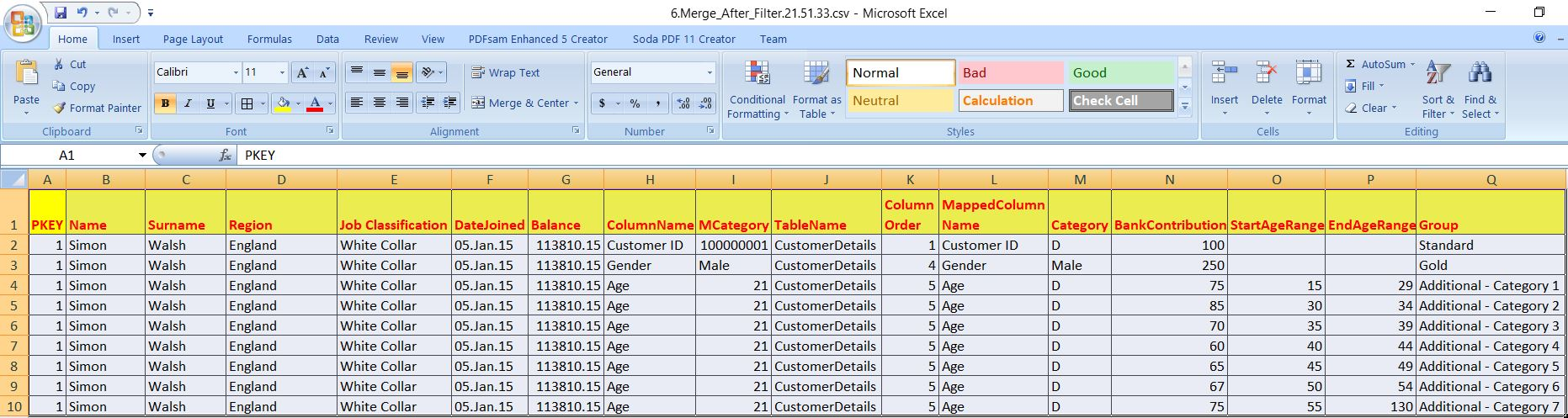`# Identifying Integer Column for next stepdf_fin2['Catg'] = df_fin2.apply(lambda row: self.getIntVal(row), axis=1)df_fin2['StAge'] = df_fin2.apply(lambda row: self.getNaN2Zero_StartAgeRange(row), axis=1)df_fin2['EnAge'] = df_fin2.apply(lambda row: self.getNaN2Zero_EndAgeRange(row), axis=1)# Dropping the old Columnsdf_fin2.drop(['Category'], axis=1, inplace=True)df_fin2.drop(['StartAgeRange'], axis=1, inplace=True)df_fin2.drop(['EndAgeRange'], axis=1, inplace=True)# Renaming the new columnsdf_fin2.rename(columns={'Catg': 'Category'}, inplace=True)df_fin2.rename(columns={'StAge': 'StartAgeRange'}, inplace=True)df_fin2.rename(columns={'EnAge': 'EndAgeRange'}, inplace=True)`

Now, the application will remove NaN from these key columns for important upcoming step.

After this step, the new data looks like –So, now, it will be easier to filter out these data based on age range against customer age int the next step as follows –

`# Handling special cases when Category from source & lookup file won't match# alternative way to implement left outer join due to specific data scenariosdf_fin2['Flag'] = np.where(((df_fin2.StartAgeRange == 0) | (df_fin2.EndAgeRange == 0)) |                           (((df_fin2.StartAgeRange > 0) & (df_fin2.EndAgeRange > 0)) &                            ((df_fin2.Category >= df_fin2.StartAgeRange)                              & (df_fin2.Category <= df_fin2.EndAgeRange))), 'Y', 'N')`

After this, new data looks like –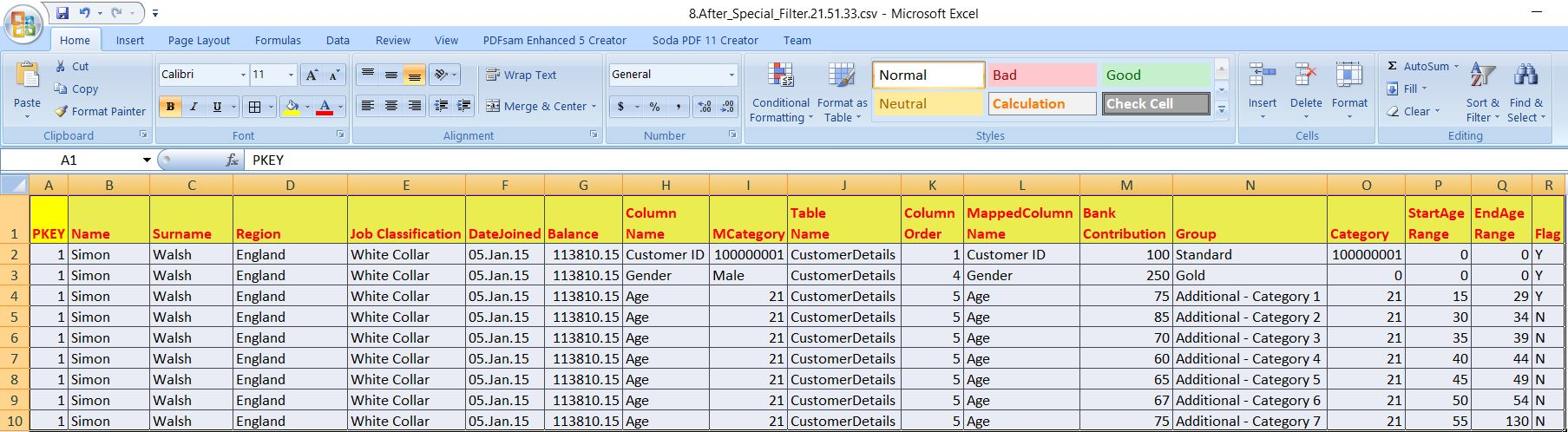Finally, filter out only records with ‘Y’. And, the data looks like as follows –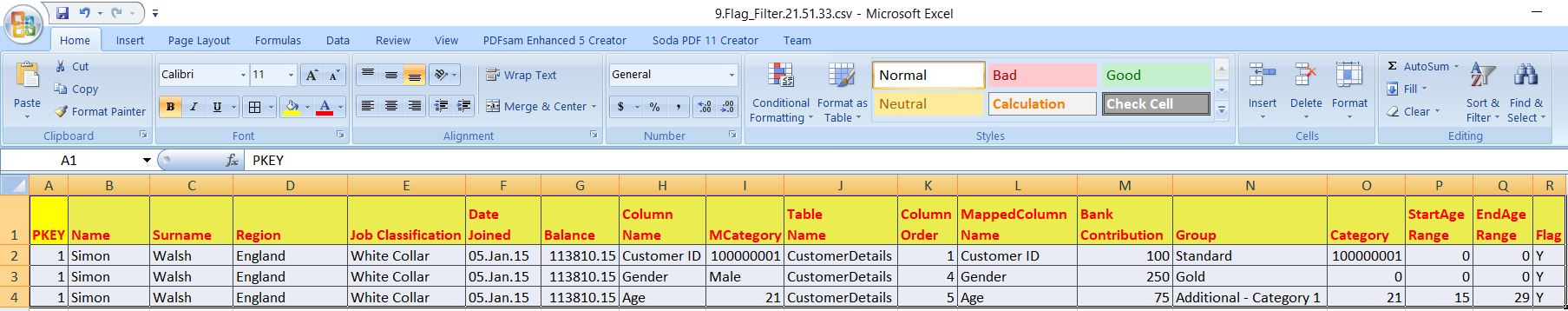Now, the application needs to consolidate Bank Contribution, Start & End Age Range & needs to re-pivot the data to make it a single row per customer. The data should look like this –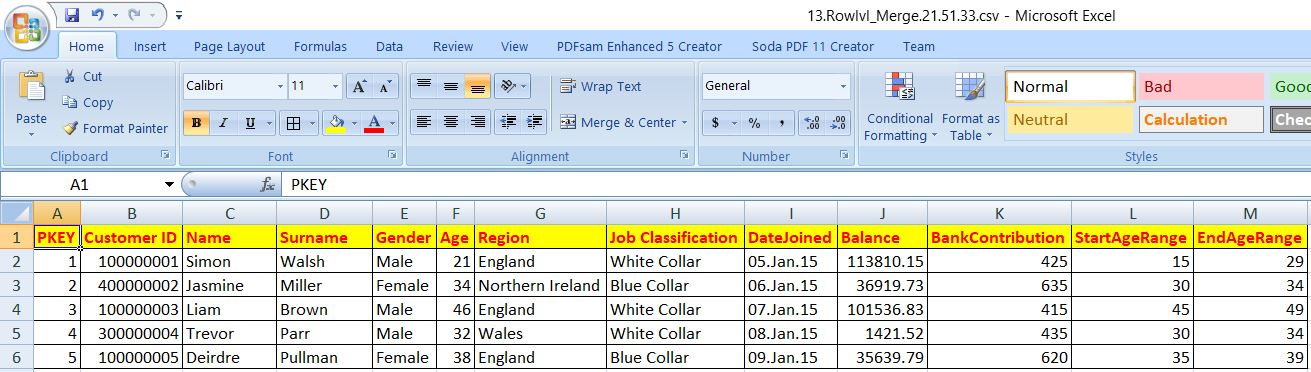Once this is done, our application is ready for all the aggregated data points.

Hence, three different categories of data transformations are self-explanatory –

Data Point – 1:

`################################################################## Country & Gender wise Bank's Contribution           ###################################################################dfCountryGender = df_rowlvl[['Region', 'Gender', 'BankContribution']]grouped_CG = dfCountryGender.groupby(['Region', 'Gender'])dCountryGen = grouped_CG.aggregate(np.sum)print("-" * 60)print("Country & Gender wise Bank's Contribution")print("-" * 60)print(dCountryGen)clog.logr('15.dCountryGen' + var + '.csv', Ind, dCountryGen, subdir)##################################################################### End Of Country & Gender wise Bank's Contribution  #####################################################################`

Data Point – 2:

`################################################################## Country & Job wise Bank's Contribution              ###################################################################dfCountryJob = df_rowlvl[['Region', 'Job Classification', 'BankContribution']]grouped_CJ = dfCountryJob.groupby(['Region', 'Job Classification'])dCountryJob = grouped_CJ.aggregate(np.sum)print("-" * 60)print("Country & Job wise Bank's Contribution")print("-" * 60)print(dCountryJob)clog.logr('16.dCountryJob' + var + '.csv', Ind, dCountryJob, subdir)##################################################################### End Of Country & Job wise Bank's Contribution     #####################################################################`

Data Point – 3:

`################################################################## Country & Age wise Savings & Bank's Contribution    ###################################################################dfCountryAge = df_rowlvl[['Region', 'StartAgeRange', 'EndAgeRange', 'Balance', 'BankContribution']]dfCountryAge['SavingsAmount'] = dfCountryAge.apply(lambda row: self.getSavingsAmount(row), axis=1)grouped_CA = dfCountryAge.groupby(['Region', 'StartAgeRange', 'EndAgeRange'])dCountryAge = grouped_CA.aggregate(np.sum)print("-" * 60)print("Country & Job wise Bank's Contribution")print("-" * 60)print(dCountryAge)clog.logr('17.dCountryAge' + var + '.csv', Ind, dCountryAge, subdir)################################################################## End Of Country & Age wise Savings & Bank's          ######### Contribution                                        ###################################################################`

Finally, these datasets will invoke an excel generator function to capture all these data into different sheets & beautify the report are as follows –

`################################################################### Writing to Excel File with Different Tabular Sheet ###################################################################dfs = [dCountryGen, dCountryJob, dCountryAge]sheets = ['Country-Gender-Stats', 'Country-Job-Stats', 'Country-Age-Stats']x = self.dfs_tabs(dfs, sheets, report_path+tgtFileName + var + '.xlsx')###################################################################             End Of Excel Sheet Writing             ###################################################################`

Key snippets from this function –

`writer = p.ExcelWriter(file_name, engine='xlsxwriter')`

This step will initiate the excel engine.

`for dataframe, sheet in zip(df_list, sheet_list):    number_rows = int(dataframe.shape)    number_cols = int(dataframe.shape)`

In this step, the application will unpack one by one sheet & produce the result into excel.

`if cnt == 0:    dataframe.to_excel(writer, sheet_name=sheet, startrow=7, startcol=5)else:    dataframe.to_excel(writer, sheet_name=sheet, startrow=5, startcol=0)`

In this step, this will create the data starting from row 7 into the first sheet, whereas the remaining two sheets will capture data from row 5.

`worksheet.set_column('A:E', 4)worksheet.set_column('F:F', 20)worksheet.set_column('G:G', 10)worksheet.set_column('H:J', 20)`

This will set the length of these columns.

`# Insert an Imageworksheet.insert_image('E1', 'Logo.png', {'x_scale':0.6, 'y_scale':0.8})`

In this case, the application will insert my blog logo on top of every page of this excel.

`# Add a number format for cells with money.money_fmt = workbook.add_format({'num_format': '\$#,##0', 'border': 1})worksheet.set_column('H:H', 20, money_fmt)`

Also, for the column with monetary information, it will generate a specific format.

`# Define our range for color formattingcolor_range = "F9:F{}".format(number_rows * 2 + 1)# Add a format. Red fill with the dark red textred_format = workbook.add_format({'bg_color':'#FEC7CE', 'font_color':'#0E0E08', 'border':1})# Add a format. Green fill with the dark green textgreen_format = workbook.add_format({'bg_color': '#D0FCA4', 'font_color': '#0E0E08', 'border': 1})# Add a format. Cyan fill with the dark green textmid_format = workbook.add_format({'bg_color': '#6FC2D8', 'font_color': '#0E0E08', 'border': 1})# Add a format. Other fill with the dark green textoth_format = workbook.add_format({'bg_color': '#AFC2D8', 'font_color': '#0E0E08', 'border': 1})worksheet.conditional_format(color_range, {'type':'cell',                                           'criteria':'equal to',                                           'value':'"England"',                                           'format': green_format})worksheet.conditional_format(color_range, {'type': 'cell',                                           'criteria': 'equal to',                                           'value': '"Northern Ireland"',                                           'format': mid_format})worksheet.conditional_format(color_range, {'type': 'cell',                                           'criteria': 'equal to',                                           'value': '"Scotland"',                                           'format': red_format})worksheet.conditional_format(color_range, {'type': 'cell',                                           'criteria': 'equal to',                                           'value': '"Wales"',                                           'format': oth_format})`

In this step, the application will color-code individual start cell to highlight specific category for better decision making visually.

4. callPivotLookUp.py (This script will call the main pivot script & process the data as per business requirement. Hence, the name comes into the picture.)

 ``` 1 2 3 4 5 6 7 8 9 10 11 12 13 14 15 16 17 18 19 20 21 22 23 24 25 26 27 28 29 30 31 32 33 34 35 36 37 38 39 40 41 42 43 44 45 46 47 48 49 50 51 52 53 54 55 56 57 58 59 60 61 62 63 64 65 66 67 68 69 70 71 72 73 74 75 76 77 78 79 80 81 82 83 84``` ```##################################################### ### Objective: Purpose of this Library is to call ### ### the parse_and_write_csv method to produce the ### ### tokenized columns based on the look-up file. ### ### ### ### Arguments are as follows: ### ### Source File, Target File & Lookup Files. ### ### ### ##################################################### import clsPivotLookUp as ct from clsParam import clsParam as cf import sys import pandas as p import clsLookUpDataRead as cl def main(): print("Calling the custom Package..") cnt_lkp = 0 try: #Default Look up table Lkp_Filename = cf.config['LKP_FILE'] # Adding New DB Table for Lookup x = cl.clsLookUpDataRead(Lkp_Filename) df_lkpF = x.ReadTable() cnt_lkp = df_lkpF.shape if cnt_lkp > 0: df_lkpF_copy = df_lkpF.copy() # Getting all the unique file names df_list_F1 = list(df_lkpF_copy['TableName'].drop_duplicates()) # File list which has Tokenization df_lkpF_Int = df_lkpF[(df_lkpF['Group'].str.len() >= 1)] df_list_F2 = list(df_lkpF_Int['TableName'].drop_duplicates()) for i in df_list_F1: if i in df_list_F2: try: inputFile = i print("*"*30) print("Reading from " + inputFile + ".csv") print("*" * 30) srcFileName = inputFile tarFileName = srcFileName + '_processed' x = ct.clsPivotLookUp(srcFileName, tarFileName, df_lkpF) ret_val = x.parse_and_write_csv() if ret_val == 0: print("Writing to file -> (" + tarFileName + ".csv) Status: ", ret_val) else: if ret_val == 5: print("File IO Error! Please check your directory whether the file exists with data!") else: print("Data Processing Issue!") print("*" * 30) print("Operation done for " + srcFileName + "!") print("*" *30) except Exception as e: x = str(e) srcFileName = inputFile print('Check the status of ' + srcFileName + ' ' + x) else: pass else: print("No Matching Data to process!") except Exception as e: x = str(e) print(x) print("No Matching Data to process!") if __name__ == "__main__": main() ```

And, the key snippet from here –

`# Getting all the unique file namesdf_list_F1 = list(df_lkpF_copy['TableName'].drop_duplicates())# File list which has Tokenizationdf_lkpF_Int = df_lkpF[(df_lkpF['Group'].str.len() >= 1)]df_list_F2 = list(df_lkpF_Int['TableName'].drop_duplicates())`

This will identify all the source files, which as similar kind of cases & process them one by one.

`x = ct.clsPivotLookUp(srcFileName, tarFileName, df_lkpF)ret_val = x.parse_and_write_csv()if ret_val == 0:    print("Writing to file -> (" + tarFileName + ".csv) Status: ", ret_val)else:    if ret_val == 5:        print("File IO Error! Please check your directory whether the file exists with data!")    else:        print("Data Processing Issue!")`

This will call the main application class & based on the return result – it will capture the status of success or failure.

Let’s check the directory of both the Windows & MAC.

Windows:MAC: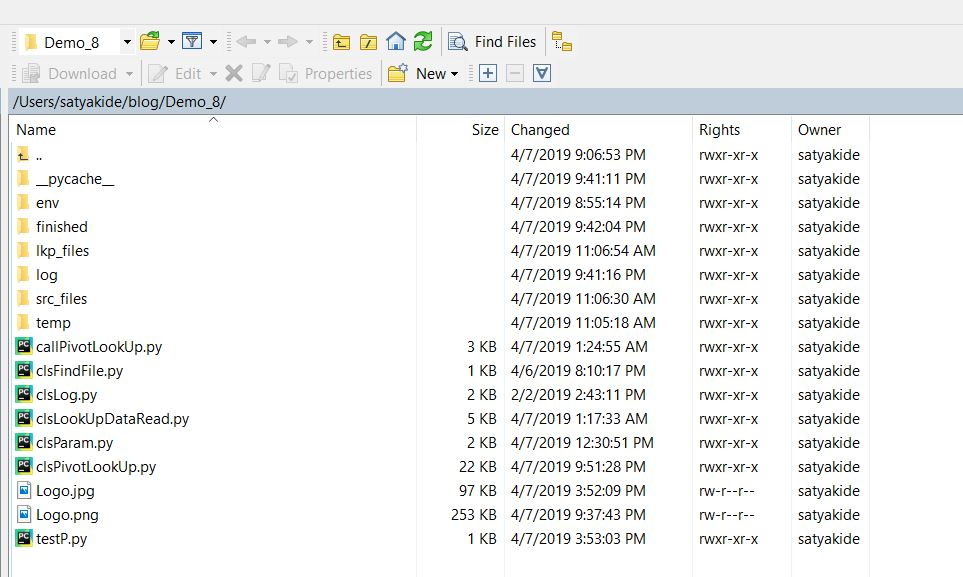Let’s check the run process –

Windows: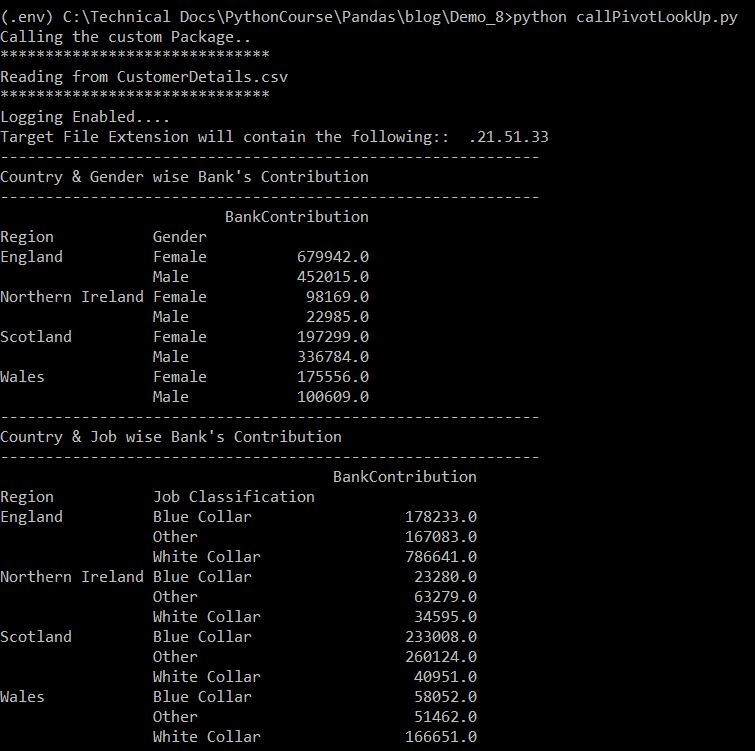MAC: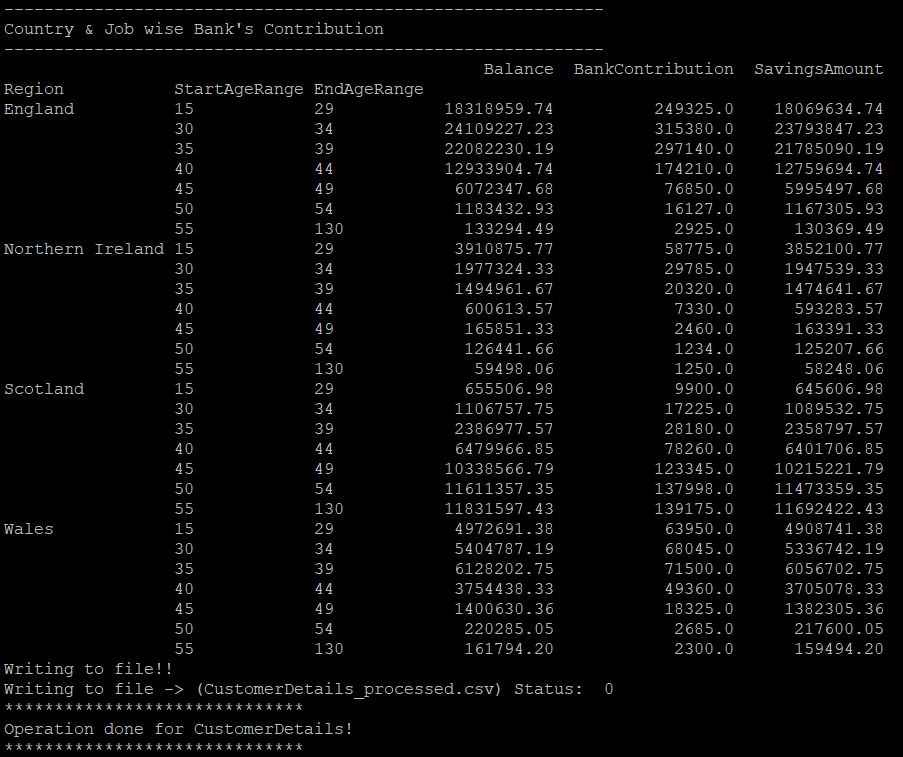Let’s see – how it looks in Excel –

Windows: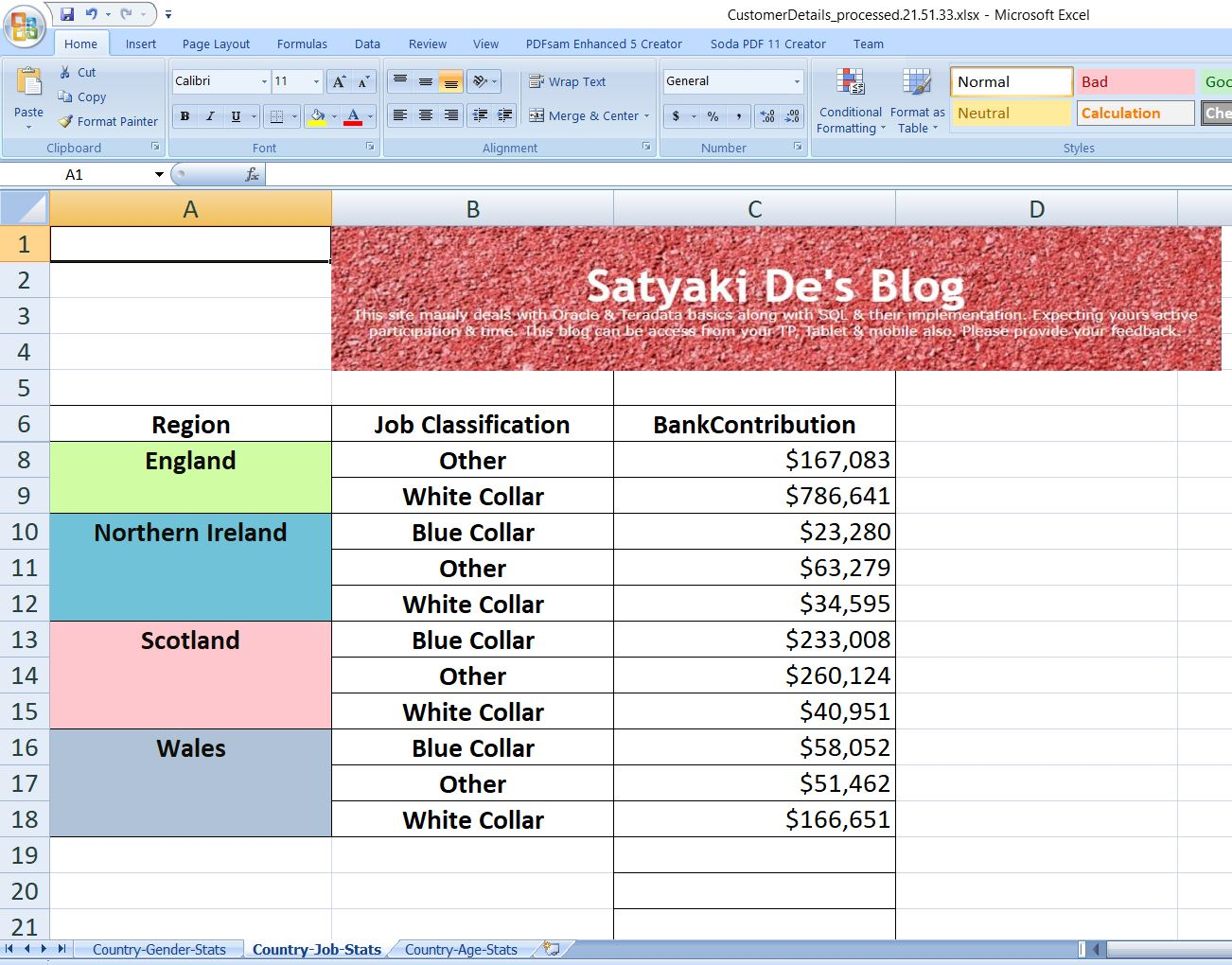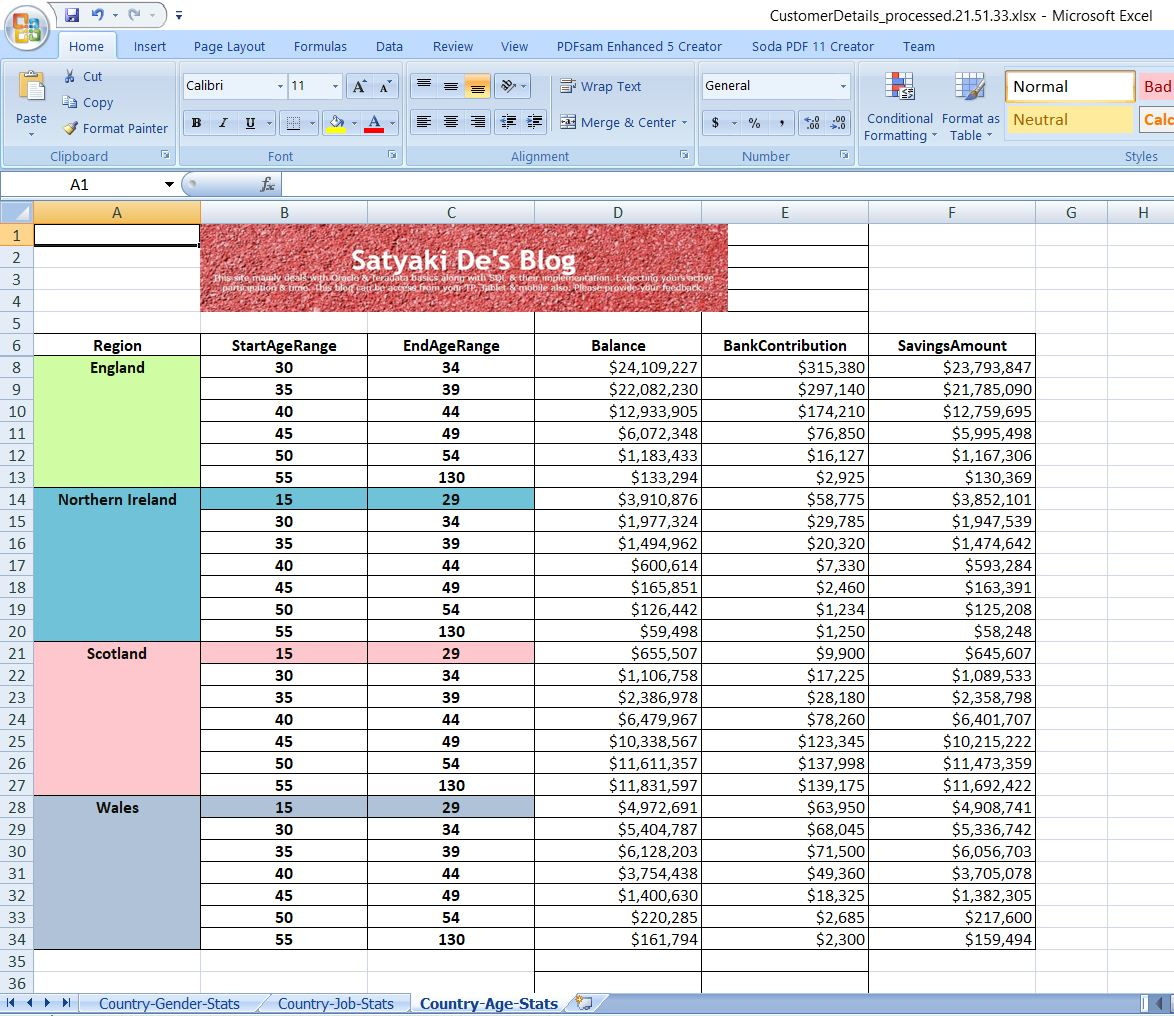MAC: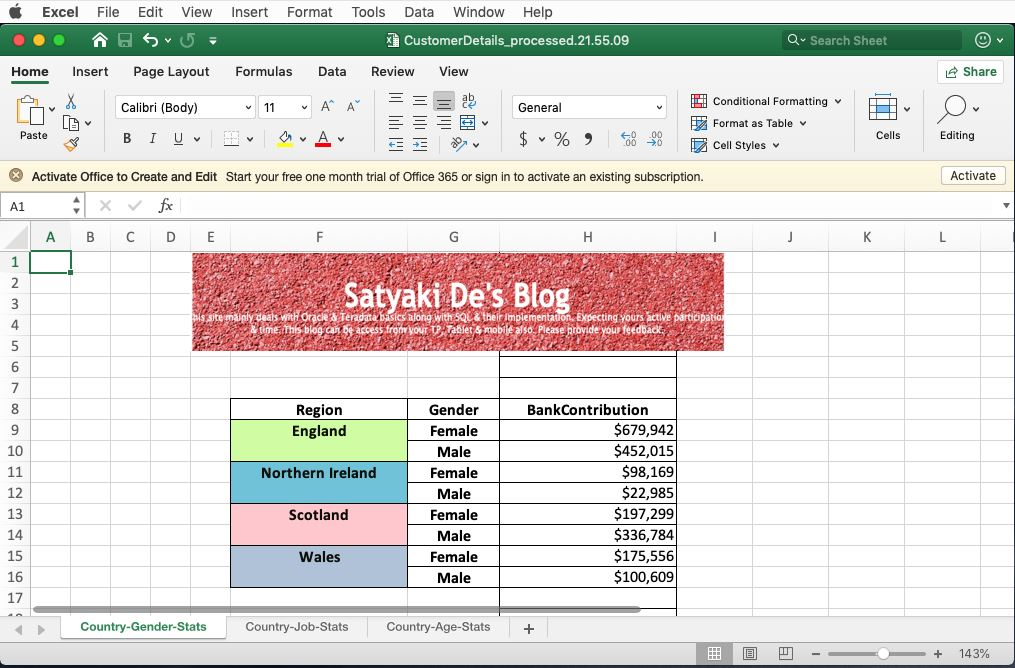So, finally, we’ve achieved our target.

Horray! We’ve done it! 😀

I hope you’ll like this effort.

Wait for the next installment. Till then, Happy Avenging. 🙂

[Note: All the sample data are available in public domain for research & study.]

## Zoho BI Reports

Here is one sample Zoho Bi Reports which directly connects with my local Oracle DB. And, as soon as I’m changing the data – it will directly reflecting the same here.

I’m sure you are really want to know the secret behind this.

Explaining the same very soon.

Bye.